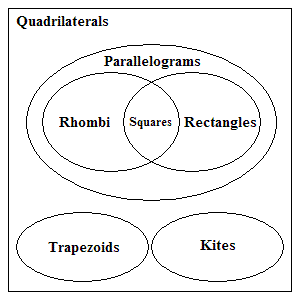A quadrilateral is a polygon with four sides.

There are many special types of quadrilateral.

A parallelogram is a quadrilateral in which both pairs of opposite sides are parallel .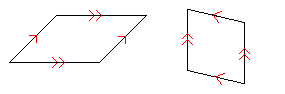A parallelogram also has the following properties:

• Opposite angles are congruent;
• Opposite sides are congruent;
• The diagonals bisect each other.

A rectangle is a parallelogram with four right angles, so all rectangles are also parallelograms and quadrilaterals. On the other hand, not all quadrilaterals and parallelograms are rectangles.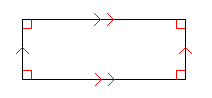A rectangle has all the properties of a parallelogram, plus the following:

• The diagonals are congruent.

A rhombus is a parallelogram with four congruent sides. The plural of rhombus is rhombi . (I love that word.)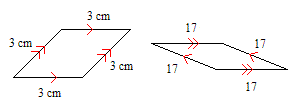A rhombus has all the properties of a parallelogram, plus the following:

• The diagonals intersect at right angles.

A square can be defined as a rhombus which is also a rectangle – in other words, a parallelogram with four congruent sides and four right angles.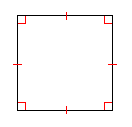A trapezoid is a quadrilateral with exactly one pair of parallel sides. (There may be some confusion about this word depending on which country you're in. In India and Britain, they say trapezium ; in America, trapezium usually means a quadrilateral with no parallel sides.)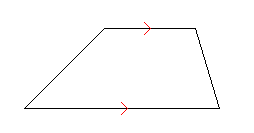An isosceles trapezoid is a trapezoid whose non-parallel sides are congruent.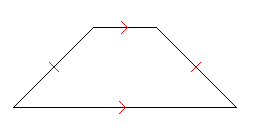A kite is a quadrilateral with exactly two pairs of adjacent congruent sides. (This definition excludes rhombi. Some textbooks say a kite has at least two pairs of adjacent congruent sides, so a rhombus is a special case of a kite.)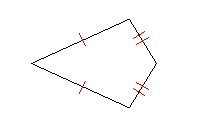A scalene quadrilateral is a four-sided polygon that has no congruent sides. Three examples are shown below.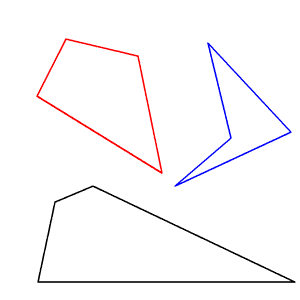## Venn Diagram of Quadrilateral Classification

The following Venn Diagram shows the inclusions and intersections of the various types of quadrilaterals.